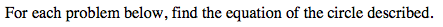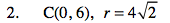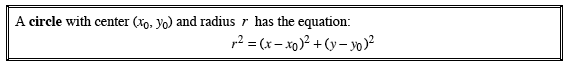### Home > PC > Chapter 13 > Lesson 13.1.1 > Problem13C-2

13C-2.x0 = 0y0 = 6

$(4\sqrt{2})^2=(\textit{x}-0)^2+(\textit{y}-6)^2$